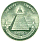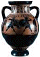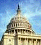xNewton's laws of motion
Posts  1 - 3  of  3trmichaels
Does Newton
replied to:  trmichaelscoffeym
Replied to:  Does Newton
The derivation for force was resulted from Newton
replied to:  coffeymjstack
Replied to:  The derivation for force was resulted from Newton
The formula Force = Mass into Acceleration